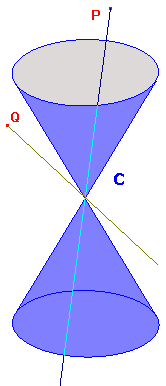the interior and exterior of a projective conic

 In RP2, a projective conic C can be viewed as a cone with vertex O, and a p-point P as a line through O. Intuitively, P lies inside C if the angle the line makes with the axis is less than the semi-vertical angle of the cone, and outside otherwise. The sketch on the right shows P inside the cone, and Q outside. Unfortunately, neither the axis nor the semi-vertical angle are preserved by projective transformations. The latter is obvious, since all cones are projective congruent. The former will become clear when we see that it is possible to map C to C in such a way that a given interior p-point maps to any other interior p-point. We require a characterization in terms of projective concepts. We begin by looking at alternative intuitive descriptions, and derive a suitable formal definition. First of all, we note that a p-line meets a p-conic at most twice, since the p-line has a linear equation and the p-conic a quadratic equation. Thus the p-line is a tangent if it meets the p-conic in just one p-point. If P is a p-point lying inside C in the above sense, then any p-line which passes through P meets C twice. It follows from the above remark that there are no tangents to C through P. If Q is a p-point lying outside C in our intuitive sense, then it is clear that there are p-lines through Q which meet C twice, and p-lines which do not meet C. By considering a p-line (a plane) rotating round Q, we see that there are two tangents through Q - one where the p-line first meets C, and one where it meets it for the last time. We believe that this is intuitive, but we will give a formal proof later.Definition Suppose that C is a p-conic, and P a p-point. Then P lies inside C if there is no tangent to C through P, and P lies outside C if there are (at least) two tangents to C through P. Of course, if a p-point T lies on C, then there is one tangent through T, namely the tangent at T. The Corollary to the Invariance Theorem shows that for any projective transformation t, maps tangents to C through P correspond to the tangents to t(C) through t(P). Thus, the number of tangents is the same for P and for t(P). This proves The interior-exterior theorem of projective geometry If C is a p-conic and t a projective transformation, then t maps the interior of C to the interior of t(C), and the exterior of C to the exterior of t(C). We now require one of the most significant results of projective geometry. This allows us to describe the interior and exterior of a p-conic in terms of polars. La Hire's Theorem If C is a p-conic, and P, Q are p-points, then Q lies on the polar of P if and only if P lies on the polar of Q. Note that, if T lies on the p-conic C, then the polar of T with respect to C is the tangent at T. Now suppose that the tangent at T passes through the p-point P. Then P lies on the polar of T, so, by La Hire's theorem, T lies on the polar of P. But T lies on C, so the polar of P meets C - at T. Immediately, we see that, if P lies inside C, then there are no tangents through P, so that the polar of P does not meet C. If there is one tangent through P, then the polar of P meets C just once, i.e. is a tangent to C, at T, say. The polar of T is this same tangent, so we must have P=T, i.e. P lies on C. Finally, suppose that there are at least two tangents through P, and that two of them meet C at S and T. Then the polar of P meets C at S and T. The polar is a p-line, so meets C at most twice. These remarks justify the earlier assertion that a p-point outside C lies on exactly two tangents. They also prove the polar characterization of interior and exterior Suppose that C is a p-conic, and that L is the polar of the p-point P. Then P lies inside C if and only if L does not meet C, P lies on C if and only if L meets C once, and P lies ouside C if and only if L meets C twice. We can also characterize the interior in terms of the equation of the projective conic. Since this leads us in a new direction, we shall give it on a separate page. We are now in a position to look at the effect of projective symmetries of a p-conic on the interior and exterior. We will get a two-point theorem, but first, we must look at the projective symmetry group of a conic.